# The Physical Basis of Time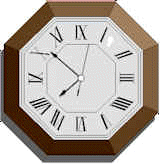you can generalize a measurement using   a set of properties that you'd like to quantify: S = {p0,p1, p2...pn} then the general expression for  a   measurement is: M=pi/p0

Imagine for a moment that your watch reads 1:00 PM and that at this instant, you turn off all time.  (reminds me of that Twilight Zone episode...)   Obviously, not something that we can actually do but... If it were possible, the measurable advance of time would cease.  All clocks would read 1:00 until  you re-started time. There is a question here though, why should the motion of a clock be any different from any other motion?  Indeed, if you could stop all time, then all motion should stop.  Kind of makes you go hmmmm.   don't it!.

Einstein was once said that "time is what a clock measures".  Not very informative -- right?   What you'd really want to know is "how does a clock measure time?"  Maybe it doesn't!  Maybe a clock measures motion!

Well, lets ask: What defines a clock?  Think for a minute about how our early ancestors kept time.   Their timepieces were the sun, the moon and the stars.  No maser clocks, no gears, no back-lit LCD displays and no numbers.   Did the stars measure time?  No.  But our mega-great grand folks could and did use the motion of the sun/moon/stars to tell what "time" it was.  Where was the measuring?   How would you or anyone else use this astro-clock?  Simple! If the sun was straight up then eat lunch.  If the sun went behind a mountain -- go to bed.  Notice that you don't say "when" the sun is straight up.

Many of our ancestors "motions" were coordinated by the motions of the sun/moon/stars.  Some motions were coordinated with respect to the motions of rocks or spears.  Why should motion of the sun/moon/stars be special?  Short answer: Its just motion but it is a little more consistent than the motion of other objects.  Or, you might say that the positions of certain astral bodies are highly coorelated to other important events.  Night, day, spring, fall, lunch etc. for example.

Today our clocks (and calendars)  represent (are models of) the motions of sun/moon/stars.  That's all -- just the motion.  We've embellished it a bit by adding the numbers.  And at first we kind of screwed it up (see Leap Years).

A clock gives us a reference motion that we can use  like we use a ruler (see "The Universe On Its Terms") to measure other motion.  For example I can express a measurement of a change in position as v=dx/dt where v is the measured change, dx is the distance traveled and dt is the reference change in position.  Yep, v is just velocity.   We've just assigned a different name to dt.   Remember that our general expression for a measurement of length is = (target)/(reference).  Here we are measuring a change in position with a reference change in position.

Of course we can use dt to measure other changes as well.  If, for example, you are pouring marbles into a bowl you can use dt as a more general reference in change to express the number of marbles per unit time.  This, of course  is pretty obvious, but the huge number of uses for clocks makes time a "noisy" subject.   There is the arrow of time, the beginning of time, the fourth dimension, time travel, thermodynamics, chaos, strings...  Too much!  The difficultiy with the subject of time is the focus on the subject of time rather than on clocks.  Time misdirects your attention like a magician uses misdirection to distract you from his slight of hand.  When the misdirection works you miss the trick and see "magic".   In this case the magic is the fourth dimension.

## Measuring Velocity

To measure a length we use a static (the static that we like best)   object -- a ruler.  Take the following example:

Netscape has a preference switch that turns applet support on. This applet will help a lot.

Notice that if we follow the measuring algorithm as described in "The Universe on its Terms" and define the bottom line as the ruler (defines a unit length and static) and the top line as the target that the measured length is always constant. Change the first number to 2.  Notice that the measurement of the top line via the bottom is still constant though twice as long.   You can use the stop button and step the bottom line a unit at a time and count the squares to show that the ratio of the two lines is always constant.  This tells us that, with respect to the chosen ruler, the target is also static (since we always measure the same number).  Clearly with respect to our material frame of reference, the bottom line is changing.  In other words we can express velocity without a notion of   of time.  It is easier, of course, to refer to a reference change in position simply as "time".

Because an expanding ruler is still just a ruler -- or a clock-- we can use it to measure other clocks just like we did with our static rulers.  Thus, we can use two standard clocks to find the relative scale of one with respect to the other.  Furthermore, if we know the scale of one frame with respect to another we can predict how fast a clock should run with respect to another.

## The Math

Now, using the scale derived on the previous page we have two new equations we can write:

s0v0 = s1v1
s0t0 = s1t1

where s0 expresses the scale of frame 0;  v0 is the reference velocity of frame 0 with respect to frame 1 and s1 is the scale of frame 1 with respect to frame 0, and v1 is the reference velocity of frame 1 with respect to frame 0.  The t's represent the reference motion or elapsed time in frame 0 and 1 as indicated by the subscripts.

The quantity (scale)(velocity) represents the "amount of space traveled".  That is, if I travel a compressed distance equal to dx I can find the amount of space traveled if I mulitply by its linear density (scale).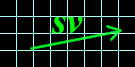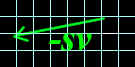SV represents the amount of space traversed and is always equal  to and opposite that of any other frame.

Now, if we apply Gallileo's relativity... If I take a step of an "amount of space" equal to SV.  This is exactly the same as you taking a step of -SV in the other frame.  If, however, your frame's scale is s1 instead of s0 (what mine is) then the equivalent "amount of space" that you travel will be -s1v1.   Even though your s1v1 will be exactly equal to s0v0.

Note that we must use only one ruler and one clock to do all the measurements from each from each frame.   Wihtout, this choice, the numbers representing the scales of other frames will differ (and confuse us).  And so to measure your scale and your velocity we use my ruler and my clock.   If, for example, I measure your contraction velocity to be ˝ mine I know that your scale must be twice as large as mine.  I can then deduce that your rulers are half as big as mine and that your clock will run half as fast as mine. (see also The Lab)

This gives us the general principle:

 For every change, there is an equal and opposite change.

Sound familiar?

(Take a look at some of the math)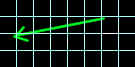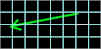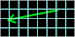In a shrinking coordinate system, it is important that the coordinates "follow" the motion of the substance which is being represented.  multiplying by the scale of the space "conserves" the quantity of the contracting space.  That is, the quantities (scale)(distance), (scale)(velocity) and (scale)(acceleration) represent  quantities that are conserved with respect to contraction and expansion.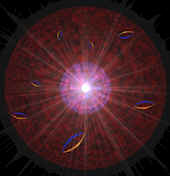An artists impression of an electron.  Courtesy of AIP.

## Representing the unseen

If an electron were to choose a ruler how big with respect to your rulers would it be?  How fast would an electron's clock run? (but electrons don't have clocks?!)  Is an electron a contracted space?  Can we measure space with space?  With respect to physics, these are important questions since the answers tell us a lot about the static and dynamic geometry of our universe. So hang on, there's...

### More to come:

 A natural unit length for electrons and space conservative laws Lorentz's scale Minkowski's space Acceleration.